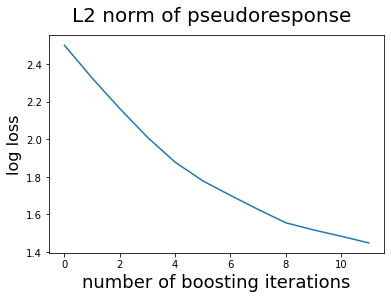This article was first published on T. Moudiki's Webpage - Python , and kindly contributed to python-bloggers. (You can report issue about the content on this page here)

Disclaimer: I have no affiliation with the scikit-learn team.

Thanks to inheritance, nnetsauce and mlsauce models share a lot of properties with scikit-learn’s Statistical/Machine learning (ML) models. That’s to say: if you’re already familiar with scikit-learn, you won’t have to spend a lot of time figuring out how do nnetsauce and mlsauce work.

nnetsauce and mlsauce notably possess methods `fit` (for training the model) and `predict` (for model testing on unseen data). And as a result, they share with scikit-learn ML models the ability to be calibrated through existing scikit-learn cross-validation functions. nnetsauce and mlsauce aren’t reinventing the wheel.

In this post, I’ll be using scikit-learn’s `GridSearchCV` on mlsauce’s LSBoostClassifier. `GridSearchCV` computes cross validation accuracy, on all the possible combinations of a grid of hyperparameters (these are the model’s free parameters, which can drive its accuracy upward or downward). Eventually, `GridSearchCV` returns the best model on the grid, with the highest accuracy, and the associated best hyperparameters.

We start by installing mlsauce:

```!pip install mlsauce
```

Then, the packages necessary for the demo:

```import mlsauce as ms
import numpy as np
import matplotlib.pyplot as plt
from sklearn.model_selection import train_test_split
from sklearn.model_selection import GridSearchCV

# splitting the data into training and testing sets
X_train, X_test, y_train, y_test = train_test_split(X, y, test_size=0.2,
random_state=1234)
```

Here is how to carry out a grid search, for a 5-fold cross-validation on
`LSBoostClassifier`:

```# hyperparameters values forming the grid:
# - "learning_rate": controls how fast the learning of residuals goes
# - "n_hidden_features": number of hidden nodes in base learners (ridge regression on nonlinear features)
# - "reg_lambda": regularization parameter in base learners (ridge regression on nonlinear features)
# - "col_sample": increases diversity of the base learners in training
# - "tolerance": controls early stopping in the learning of residuals

parameters = {
"learning_rate": [0.001, 0.005, 0.01, 0.05, 0.1, 0.2, 0.3],
"n_hidden_features": [25, 50, 60, 70, 80, 90],
"reg_lambda": [0.1, 0.2, 0.3, 0.4, 0.5],
"col_sample": [0.3, 0.4, 0.5, 0.6],
"tolerance": [1e-5, 1e-4, 1e-3, 1e-2, 0.1, 0.2]
}

# n_estimators is the number of steps in the learning descent
regr = GridSearchCV(ms.LSBoostClassifier(n_estimators=200),
scoring='accuracy',
param_grid=parameters,
cv=5, verbose=3, n_jobs=-1)
```
```regr.fit(X_train, y_train)
```
```Fitting 5 folds for each of 5040 candidates, totalling 25200 fits

[Parallel(n_jobs=-1)]: Using backend LokyBackend with 2 concurrent workers.
[Parallel(n_jobs=-1)]: Done  30 tasks      | elapsed:    4.1s
[Parallel(n_jobs=-1)]: Done 318 tasks      | elapsed:   31.9s
[Parallel(n_jobs=-1)]: Done 638 tasks      | elapsed:  1.2min
[Parallel(n_jobs=-1)]: Done 1006 tasks      | elapsed:  2.1min
[Parallel(n_jobs=-1)]: Done 1579 tasks      | elapsed:  3.7min
[Parallel(n_jobs=-1)]: Done 2038 tasks      | elapsed:  4.9min
[Parallel(n_jobs=-1)]: Done 2674 tasks      | elapsed:  6.9min
[Parallel(n_jobs=-1)]: Done 3544 tasks      | elapsed:  9.0min
[Parallel(n_jobs=-1)]: Done 4488 tasks      | elapsed: 11.4min
[Parallel(n_jobs=-1)]: Done 5660 tasks      | elapsed: 13.9min
[Parallel(n_jobs=-1)]: Done 6932 tasks      | elapsed: 16.7min
[Parallel(n_jobs=-1)]: Done 8066 tasks      | elapsed: 19.9min
[Parallel(n_jobs=-1)]: Done 9318 tasks      | elapsed: 23.2min
[Parallel(n_jobs=-1)]: Done 10678 tasks      | elapsed: 26.7min
[Parallel(n_jobs=-1)]: Done 12394 tasks      | elapsed: 30.6min
[Parallel(n_jobs=-1)]: Done 14050 tasks      | elapsed: 34.7min
[Parallel(n_jobs=-1)]: Done 15426 tasks      | elapsed: 39.0min
[Parallel(n_jobs=-1)]: Done 17116 tasks      | elapsed: 43.5min
[Parallel(n_jobs=-1)]: Done 19314 tasks      | elapsed: 48.4min
[Parallel(n_jobs=-1)]: Done 21206 tasks      | elapsed: 54.0min
[Parallel(n_jobs=-1)]: Done 23140 tasks      | elapsed: 59.5min
[Parallel(n_jobs=-1)]: Done 25200 out of 25200 | elapsed: 64.7min finished
6%|▌         | 12/200 [00:00<00:00, 252.36it/s]

GridSearchCV(cv=5, error_score=nan,
estimator=LSBoostClassifier(activation='relu', backend='cpu',
learning_rate=0.1, n_estimators=200,
n_hidden_features=5, reg_lambda=0.1,
row_sample=1, seed=123, solver='ridge',
tolerance=0.0001, verbose=1),
iid='deprecated', n_jobs=-1,
param_grid={'col_sample': [0.3, 0.4, 0.5, 0.6],
'learning_rate': [0.001, 0.005, 0.01, 0.05, 0.1, 0.2,
0.3],
'n_hidden_features': [25, 50, 60, 70, 80, 90],
'reg_lambda': [0.1, 0.2, 0.3, 0.4, 0.5],
'tolerance': [1e-05, 0.0001, 0.001, 0.01, 0.1, 0.2]},
pre_dispatch='2*n_jobs', refit=True, return_train_score=False,
scoring='accuracy', verbose=3)
```

```from time import time

start = time()
regr.best_estimator_.fit(X_train, y_train)
print("\n")
print(f"Elapsed: {time() - start}")
```
```100%|██████████| 12/12 [00:00<00:00, 249.02it/s]

Elapsed: 0.05630898475646973
```

Adjusting the model is quite fast, due to the `tolerance` hyperparameter presented before, which controls the early stopping of the learning descent – and subsequently, overfitting. 12 iterations where necessary.

Best hyperparameters found

```print(regr.best_params_)
```
```{'col_sample': 0.4, 'learning_rate': 0.2, 'n_hidden_features': 90, 'reg_lambda': 0.1, 'tolerance': 0.1}
```

In sample cross-validation accuracy (to be compared to 1.):

```print(regr.best_score_)
```
```0.9802197802197803

```

Predicting on unseen data and obtain accuracy (to be compared to 1.):

```print(regr.score(X_test, y_test))
```
```0.9385964912280702
```

To finish, this image depicts the L2 norm of successive residuals in the fitting
process (see here), and the effect of `tolerance`.

```fig = plt.figure()
plt.plot(np.log(regr.best_estimator_.obj['loss']))
fig.suptitle('L2 norm of pseudoresponse', fontsize=20)
plt.xlabel('number of boosting iterations', fontsize=18)
plt.ylabel('log loss', fontsize=16)
```As noticed before, due to the
tolerance level of `0.1`, the algorithm is stopped early, after 12 iterations, before
reaching the total budget of 200 iterations. This, of course, has an influence on
the time elapsed in the training procedure, and prevents overfitting from occurring.

```import platform

print(platform.machine())
print("\n")
print(platform.version())
print("\n")
print(platform.platform())
print("\n")
print(platform.uname())
print("\n")
print(platform.system())
print("\n")
print(platform.processor())
```
```x86_64

#1 SMP Thu Jul 23 08:00:38 PDT 2020

Linux-4.19.112+-x86_64-with-Ubuntu-18.04-bionic

uname_result(system='Linux', node='436f563181bf', release='4.19.112+', version='#1 SMP Thu Jul 23 08:00:38 PDT 2020', machine='x86_64', processor='x86_64')

Linux
x86_64
```